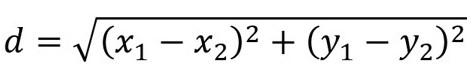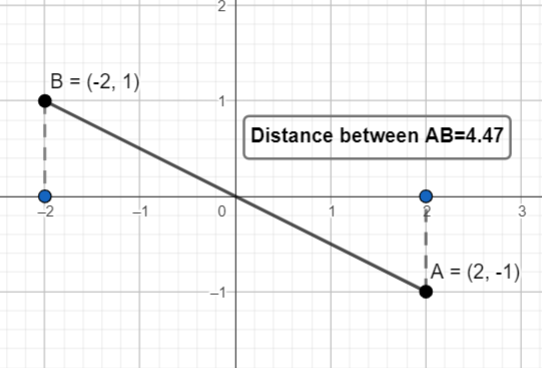Open in App
Not now

# Euclidean Distance using Scikit-Learn – Python

• Difficulty Level : Hard
• Last Updated : 25 Dec, 2022

Scikit-Learn is the most powerful and useful library for machine learning in Python. It contains a lot of tools, that are helpful in machine learning like regression, classification, clustering, etc. Euclidean distance is one of the metrics which is used in clustering algorithms to evaluate the degree of optimization of the clusters.

In geometry, we all have calculated the distance between two points using the well-known DISTANCE FORMULA in two dimensions:EUCLIDEAN DISTANCE FORMULA

where, (x1, x2) and (x2, y2) are the points on cartesian plane.Similarly, Euclidean Distance, as the name suggests, is the distance between two points that is not limited to a 2-D plane.  We can do so by using the Scikit-Learn library and importing its required directories.

## Using Scikit – Learn Euclidean Distance function

Let us consider an array of integers and we try to find their Euclidean Distance from the origin.

## Python3

 `# importing euclidean distance` `# function from scikit-learn library` `from` `sklearn.metrics.pairwise ``import` `euclidean_distances`   `# importing numpy library` `import` `numpy as np  ` `x ``=` `np.array([[``2.3``, ``1.6``, ``7.9``], ` `              ``[``0``, ``9``, ``2``], ` `              ``[``5``, ``7``, ``2.9``]])`   `# distance between X and Origin(0,0)` `distance ``=` `euclidean_distances(x, [[``0``, ``0``, ``0``]])` `print``(distance)`

Output:

```[[8.38212384]
[9.21954446]
[9.07799537]]```

Similarly, we can find Euclidean Distance between two array elements. In the below code, we have calculated the distance between each possible unique pair of points. Hence if the lists contain m and n elements respectively then we will have m * n elements in the output array.

## Python3

 `# importing euclidean distance` `# function from scikit-learn library` `from` `sklearn.metrics.pairwise ``import` `euclidean_distances`   `# importing numpy library` `import` `numpy as np  ` `X ``=` `np.array([[``2.3``, ``1.6``, ``7.9``], ` `              ``[``0``, ``9``, ``2``], [``5``, ``7``, ``2.9``]])` `Y ``=` `np.array([[``34``, ``2.9``, ``5.8``], [``4``, ``7``, ``2``],` `              ``[``6.5``, ``1``, ``0``]])`   `distance ``=` `euclidean_distances(X, Y)` `print``(distance)`

Output:

```[[31.79606894  8.17679644  8.96716232]
[34.75125897  4.47213595 10.5       ]
[29.43161565  1.3453624   6.83081254]]```

As we can see that the output is a 2D array. Each element of this array, has the distance between one point of the array, here ‘X’, to the other set of points of the second array, here ‘Y’.

#### How Euclidean distance can be used in clustering algorithms ?

Clustering algorithms are a type of unsupervised machine learning technique that involves dividing a dataset into groups (called clusters) based on their similarity. Euclidean distance is often used as a measure of similarity between data points, with points that are closer to each other being considered more similar. In a clustering algorithm, the distance between points is used to determine which points should be grouped together in the same cluster. This can be done by calculating the Euclidean distance between each pair of points and using a threshold value to determine which points should be grouped together. Alternatively, clustering algorithms can use the Euclidean distance between points to calculate the centroid of a cluster, which is the mean position of all the points in the cluster. This can be used to update the positions of the points in the cluster and improve the accuracy of the clustering algorithm.

My Personal Notes arrow_drop_up
Related Articles